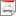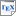[KNS03a] M. Kwiatkowska, G. Norman and J. Sproston. Symbolic Computation of Minimal Probabilistic Reachability. Technical report CSR-03-01, University of Birmingham, School of Computer Science. January 2003. [ps.gz] [pdf] [bib] Downloads:ps.gz (138 KB)pdf (352 KB)bib Links: [Google] [Google Scholar] [CiteSeer] Abstract. This paper continues our study of the verification problem for infinite-state systems featuring both nondeterministic and probabilistic choice. In an earlier paper we defined symbolic probabilistic systems, an extension of the framework of symbolic transition systems due to Henzinger et. al., and considered the problem of deciding the maximal probability of reaching a set of target states. A symbolic probabilistic system is an infinite-state system equipped with an algebra of symbolic operators on its state space, additionally extended with a symbolic encoding of probabilistic transitions to obtain a model for infinite-state probabilistic systems. In this paper we generalise the notion of symbolic probabilistic systems and consider the minimal reachability problem, that is, the problem of computing the minimal probability of reaching a given set of target states. An exact answer to this problem is obtained algorithmically via iteration of a refined version of the classical predecessor operation, combined with intersection and set difference operations. As in the previous work on symbolic transition systems, our state space exploration algorithm is semi-decidable for infinite-state systems. Together with the earlier work concerning the maximal reachability problem, the results presented here yield a semi-decidable algorithm for model checking symbolic systems against the probabilistic temporal logic PCTL. We illustrate our approach with the help of probabilistic timed automata, for which previous verification techniques suffered from an unnecessarily fine subdivisions of the state space, or which returned only estimates of the actual probabilities.